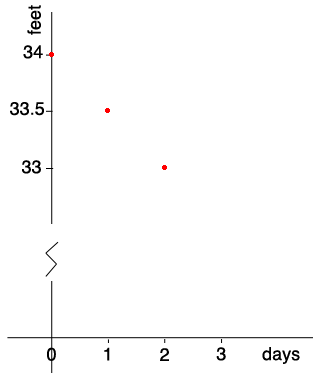SEARCH HOMEMath Central Quandaries & QueriesQuestion from emoni, a student: Suppose that the water level of a river is 34 feet and that it is receding at a rate of 0.5 foot per day.Hi Emoni,

The subject line of your email was y = mx + b so I expect you are to find the equation of the line that describes the depth of the water.

I would start by drawing a graph with the Y-axis the level of the water in feet and the X-axis the time in days. There is a break in the Y-axis so I can fit the graph on a page.The water level is 34 feet on day zero and is dropping at 0.5 feet per day so the level on day 1 is 33.5 feet and on day 2 is 33 feet. Can you write an equation for the line passing through these points, the red dots on the graph?

PennyMath Central is supported by the University of Regina and the Imperial Oil Foundation.